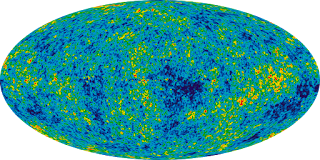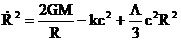## Thursday, May 3, 2018

### The standard cosmological modelMap of the Cosmic Background Radiation
In 1927, the Belgian priest and astronomer Georges Lemaître discovered Hubble’s law.
Yeah that’s right. Hubble did not discover the law until 1929. What happened was that Lemaître published it in French in a low-impact journal (Annales de la Société Scientifique de Bruxelles), while Hubble published it two years later in English in the Proceedings of The National Academy of Sciences, received much more publicity and his name got associated with the discovery.
Combined with Einstein’s cosmological equation, Lemaître-Hubble’s law implies that the universe is expanding. In an article published in 1931 in Nature, Lemaître drew the consequence by proposing the Big Bang theory, so called in derision by its opponent Fred Hoyle in 1950. The name caught on.
In 1948, Ralph Alpher, George Gamow and Robert Herman made two surprising predictions, based on the Big Bang theory: the average composition of the mass of the cosmos (three quarters hydrogen and one quarter helium), and the existence of the cosmic background radiation. Both were confirmed during the sixties. From that point, the Big Bang theory became the standard cosmological theory.
Shortly after the Big Bang, as the universe expanded, the temperature gradually became lower. While the temperature was greater than 3000 absolute degrees, all the matter of the cosmos (a mixture of hydrogen and helium) was in a plasma state, the same as the sun now, so it was opaque. When the temperature dropped below that value (a few hundred thousand years after the Big Bang) matter moved to the gaseous state and suddenly became transparent. What we see as the cosmic background radiation is just the last image of that plasma, a fraction of a second before its conversion into a gas.
From then until now the universe has continued to expand and the apparent temperature of the cosmic background radiation has gone down until today’s value, very close to absolute zero: 2.72548K. This value is practically the same in all directions of space. Only the fifth decimal (8) changes. The maps that show the radiation, with red and blue areas, use the false color technique to exaggerate the differences. Only thus can they be noticed.Power spectrum of the acoustic waves in theCosmic Background Radiation
Although the differences are so small, it is possible to distinguish the presence of acoustic waves in the cosmic background radiation. Starting from the ever improved data obtained successively by the space telescopes COBE, WMAP and Planck, cosmologists have built a mathematical model of the cosmic background radiation that depends on six fundamental parameters. These parameters are then adjusted until the power spectrum of the acoustic waves of the model corresponds as much as possible to the detected real data, as indicated in the attached figure.
This is the standard cosmological model LCDM, meaning that it includes the cosmological constant (L), and Cold Dark Matter. One of the basic points of this model is that the curvature of the universe is practically equal to zero. This means that space is flat (Euclidean) and consequently the second term of Einstein’s cosmological equation is zero. In addition, the expansion speed of the universe would have exactly the critical value, so the cosmos will expand more and more, without ever shrinking again. In fact, it appears that the expansion is accelerating, as I indicated in another post.Einstein Cosmological Equation
Of the two remaining terms in the cosmological equation, the first represents the gravitational effect of the matter in the cosmos. This term contributes 31% of the critical value to the expansion speed. The other non-zero term, that of the cosmological constant, would provide the other 69% of the critical value. The trouble is that the cosmic mass that we are able to detect, based essentially on hydrogen and helium, only equals 5% of the critical value. Therefore, there is a missing 26% that we don’t know where it comes from. We call it dark matter. The cosmological constant term, which we also don’t know what it means, is called dark energy.
The Hubble constant is not one of the six basic parameters of the standard cosmological model, but its current value H0 (which varies with time, although it is called a constant) can be inferred from these parameters. The value obtained from the satellite Planck data is 67.74±0.46.
And here we have the biggest problem detected so far in the standard cosmological model: the last direct measurement of the value of H0 from the speed of the galaxies near us, which was made public on February 22, 2018 and was obtained using the Hubble Space Telescope, yields the following result: 73.45±1.66, which is quite far from the value obtained from the standard cosmological model. Notice that the two intervals do not even overlap. This discrepancy can be resolved in three ways:
Either the data obtained in February of 2018 are wrong. They are expected to be improved so that their uncertainty will be reduced below 1%.
Or the standard cosmological model must be modified. This should be done without reducing the almost perfect adaptation of the current model to the power spectrum of the acoustic waves.
Or we should look for a different and more appropriate cosmological model. The problem is that right now we don’t know how this could be done. Perhaps we need a new Einstein.

The same post in Spanish
Thematic thread on Standard Cosmology: Preceding Next
Manuel Alfonseca